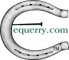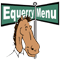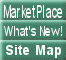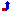[Back to Equerry Humor Barn - Horse Humor]

The well known fact that all horses have an infinite number of legs is based on the logically proven fact that all horses are the same color:

Lemma I: All horses are the same color

Proof by induction.

Base case: 1 horse is clearly the same color as itself

Assume all sets of n horses are the same color

Take a collection of n horses, they must be of the same color. Add another horse and take away a different one. Here we have a different set of n horses all of which are the same color. Thus the n+1 horses must be of the same color.

All horses are the same color by induction.

Continuing, we can then show that all horses have an infinite number of legs. Clearly horses have an even number of legs (4), but they have forelegs in front and two legs in back which makes six legs. This is an odd number of legs for a horse to have. The only number that is both odd and even is infinity. Therefore, horses must have an infinite number of legs.

To add weight to this argument we ponder whether a horse with a finite number of legs could exist. Clearly this would be a horse of a different color, but those don't exist!

To put it another way...

THE PEJORATIVE CALCULUS

Lemma 1. All horses are the same color (by induction).

Proof. It is obvious that one horse is the same color.

Let us assume the proposition P(k) that k horses are the same color and use this to imply that k+1 horses are the same color.
Given the set of k+1 horses, we remove one horse; then the remaining k horses are the same color, by hypothesis.
We remove another horse and replace the first; the k horses, by hypothesis, are again the same color.
We repeat this until by exhaustion the k+1 sets of k horses have been shown to be the same color.
It follows that since every horse is the same color as every other horse, P(k) entails P(k+1).
But since we have shown P(1) to be true, P is true for all succeeding values of k, that is, all horses are the same color.

Theorem 1. Every horse has an infinite number of legs. (Proof by intimidation.)

Proof. Horses have an even number of legs.
Behind they have two legs and in front they have fore legs.
This makes six legs, which is certainly an odd number of legs for a horse.
But the only number that is both odd and even is infinity.
Therefore horses have an infinite number of legs.

Now to show that this is general, suppose that somewhere there is a horse with a finite number of legs.
But that is a horse of another color, and by the lemma that does not exist.

QED

from A Random Walk in Science (by Joel E. Cohen?):Top